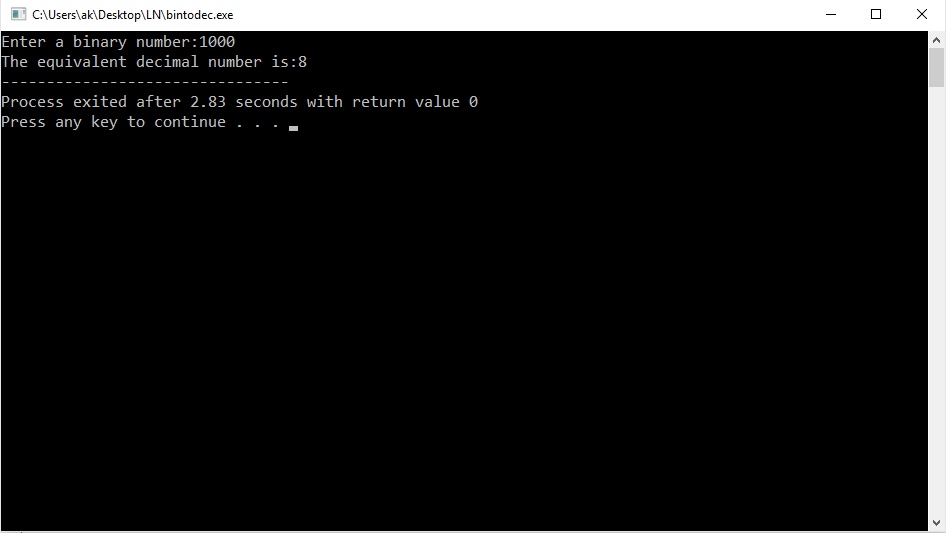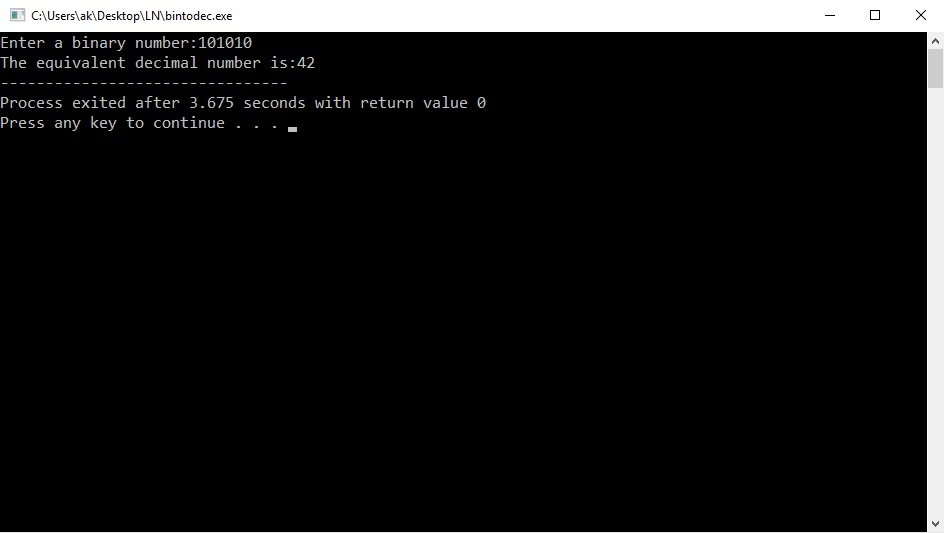# Question & Answer: Write a recursive function (with no loops or static variables) named binToDec that takes a string object as a parameter. T…..

Write a recursive function (with no loops or static variables) named binToDec that takes a string object as a parameter. The string will consist of 1s and 0s, representing a binary number. The function should return an int giving the decimal equivalent. For example,

```cout << binToDec("101010") << endl;
```

should print out 42.

Don't use plagiarized sources. Get Your Custom Essay on
Question & Answer: Write a recursive function (with no loops or static variables) named binToDec that takes a string object as a parameter. T…..
GET AN ESSAY WRITTEN FOR YOU FROM AS LOW AS \$13/PAGE

We can calculate this conversion as 1*25 + 0*24 + 1*23 + 0*22 + 1*21 + 0*20. This is, incidentally, the same as how decimal numbers work, except the base is different. For example “1027” is 1*103 + 0*102 + 2*101 + 7*100.

Notice that the indices of the characters count in the opposite direction as the exponents do.

You may not use any built-in base-conversion functionality of C++.

You may write a helper function that meets the specifications and calls your recursive function with additional arguments.

You may use the string::substr() function if you want.

#include<iostream>

#include<stdlib.h> /* header file for atoi() and itoa() functions */

#include<cmath> /* header file for pow() function */

using namespace std;

int decimal,weight; /* global variables, all the global variables have the initial value zero(0), so decimal=0, weight=0 */

int binToDec(char number) /* function definition starts here, and it is taking a string as its argument and returns an integer */

{

int bit,n; /* local variables */

char n1; /* local variable */

n=atoi(number); /* converts the string to number and storing in n */

if(n>0) /* if n is greater than 0 */

{

bit=n%10; /* identifying the right most digit */

decimal=decimal+bit*pow(2,weight); /* calculating the partial sum (by multiplying the digit with its position value in binary) */

n=n/10; /* updating the number by dividing with 10 */

weight++; /* incrementing the weight value */

itoa(n,n1,10); /* converting the integer n to string and storing in n1, 10 is the base of the number system */

binToDec(n1); /* recursive call to binToDec() */

}

else return decimal; /* returning the value of decimal to the main() function */

}

int main() /* main() function definition */

{

char number; /* string declaration */

cout<<“Enter a binary number:”;

cin>>number; /* taking a binary number into number */

cout<<“The equivalent decimal number is:”<<binToDec(number); /* function call to binToDec() */

return 0;

}

Output: